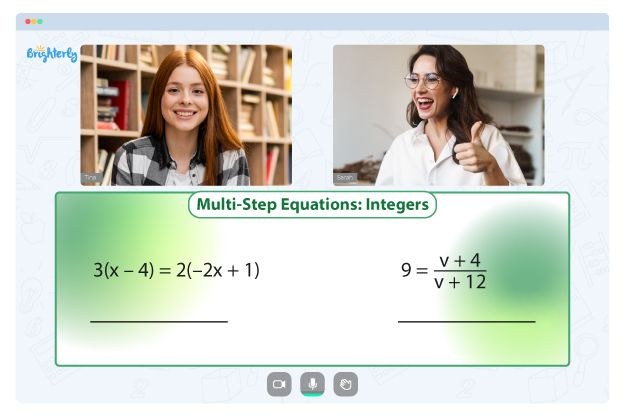# Simplifying Exponents Worksheets

Numbers increase or reduce as we work with them. So, they can become hard to keep up with in complicated math problems. Simplifying exponents allow us to work with them without worrying about losing track.

For example, when a number has to be multiplied more than twice, write it in exponents. But this concept may be challenging for grade school students, even after being taught repeatedly. Educators are introducing resources to help students understand this math concept. The new simplifying exponents worksheets can help kids learn how to write numbers in simple exponents.

## Benefits of using the simplify exponents worksheets

Here are some of the benefits of a simple exponents worksheet:

• The simple exponent worksheet teaches children how to simplify numbers using exponents so they do not get confused when dealing with math problems.
• Simplifying exponents worksheet PDF allows kids to remember the principles of simplifying exponents.
• Simplify expressions with exponents worksheets show kids how to simplify an exponent in different ways to get the same result.
• These tools help students memorize the techniques for solving exponential function problems, giving them confidence to approach any math problem with a positive mindset.

Math for Kids

Is Your Child Struggling With Math?
1:1 Online Math Tutoring### Simple Exponent Worksheet PDF

Simple Exponent Worksheet### Simple Exponents Worksheet PDF

Simple Exponents Worksheet### Simplify Exponents Worksheet PDF

Simplify Exponents Worksheet### Simplifying Exponents Worksheet PDF

Simplifying Exponents Worksheet PDF

Problems with Equations?• Does your child struggle to master of equations?
• Try learning with an online tutor.

Is your child having trouble grasping equations? An online tutor could provide the necessary assistance.